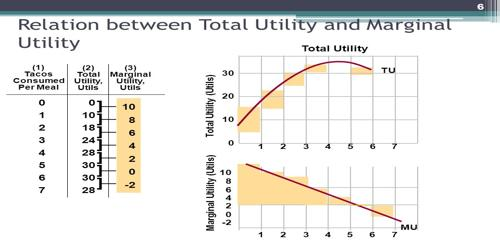Economics

# Concept of Total UtilityConcept of Total Utility

Total utility refers to the utility derived out of the consumption of a commodity. The total satisfaction received from the consumption of given quantities of a commodity by a consumer within a given time period is called total utility. In other words, total utility is the sum of all marginal utilities obtained from the consumption of different units of  a commodity. For example, suppose a consumer consumes first unit of an orange and gets 8 utils utility. As he consumes second unit of orange his total utility increases to 14 utils i.e 8+6 if he gets 6 utils utility from second orange. In the same way total utility increases to 18 utils (8+6+4) as he consumes third orange and gets 4 utils from it.

Explanation

In other words, the total satisfaction derived from the consumption of various units of goods and services is called total utility. The psychologists and economists have discovered that humans are not happy from a commodity they are using for longer period of time than comparison to the commodity they used in starting. (Using a brand new phone will make you more happy than using it after an year continuously, if a person is rational.) Every unit of a commodity has its marginal utility (a utility derived from the consumption of an additional unit), and the total utility is the summation of all these individual marginal utilities.

So what these experts did is that they noted the change in the utility. Remember these are only cardinal units which means a consumer give it some points to utility gained (it is like the one judges give some people points for the dance, music or any other talent). But these points are decreasing.

Total utility includes the satisfaction derived from a particular good or service through the duration of its lifespan. For example, a cookie provides a level of total utility as determined by its singular consumption, while a bag of cookies may provide its total utility over the course of time it takes to completely consume all of the cookies in the bag, with each cookie consumed providing a level of marginal utility contributing to the total.

For example, a person consumes eggs and gains 50 utils of total utility. This total utility is the sum of utilities from the successive units (30 utils from the first egg, 15 utils from the second and 5 utils from the third egg).

Summing up total utility is the amount of satisfaction (utility) obtained from consuming a particular quantity of a good or service within a given time period. It is the sum of marginal utilities of each successive unit of consumption.

Another example, if the 1st ice-cream gives you a satisfaction of 20 utils and 2nd one gives 16 utils, then TU from 2 ice-creams is 20 + 16 = 36 utils. If the 3rd ice-cream generates satisfaction of 10 utils, then TU from 3 ice-creams will be 20+ 16 + 10 = 46 utils.

Information Source: